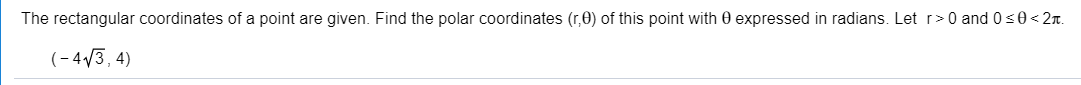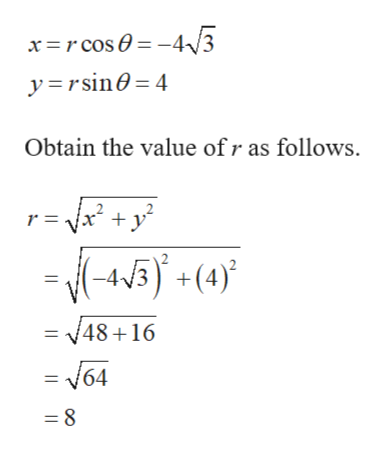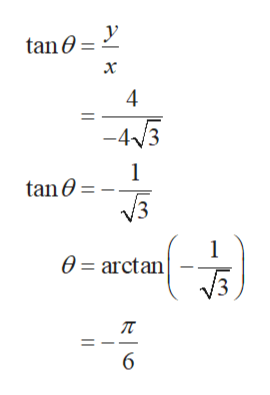The rectangular coordinates of a point are given. Find the polar coordinates (r,0) of this point with 0 expressed in radians. Let r>0 and 0 s0

Questionhelp_outlineImage TranscriptioncloseThe rectangular coordinates of a point are given. Find the polar coordinates (r,0) of this point with 0 expressed in radians. Let r>0 and 0 s0<2t. (-43, 4) fullscreen
Step 1

The given rectangular coordinates of a point is,

Step 2

Find the polar coordinates (r, θ) of this point with 0≤θ<2π.help_outlineImage Transcriptioncloseyrsin 4 Obtain the value ofr as follows. 2 r =x y (-45) +(4)} 2 = 48+16 = N64 8 fullscreen
Step 3

Obtain θ as...help_outlineImage Transcriptionclosey tan0 х -43 1 tan0 3 1 0 = arctan fullscreen

Want to see the full answer?

See Solution

Want to see this answer and more?

Our solutions are written by experts, many with advanced degrees, and available 24/7

See Solution
Tagged in

Other